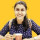1. Home >
2. Apps >
3. Groups >

# Computer Graphics Question Bank

Question asked by Ankita Katdare in #Coffee Room on Nov 6, 2010Ankita Katdare · Nov 6, 2010
Rank A1 - PRO
Following are the frequently asked questions in theory and viva of Different University Exams.

1. What is the various applications of computer graphics?
4. Write short notes on
a. Persistence
b. resolution
c. Aspect ratio
5. Define pixel.
6. What is meant by frame buffer?
7. List the important characteristics of video display device.
8. What are the two basic techniques for producing color display with a CRT?
10. Explain about flat panel displays devices.
11. List the important characteristics of hardcopy devices.
12. Differentiate LCD & LEDs.
13. List the classification of the printers.
14. Comparison between line printers and dot matrix printers.
15. Explain the features of Inkjet printer.
16. Name the input devices.
17. Compare track ball and space ball
18. Define digitizers.
20. List the advantages of the laser printer.
21. What are the different kinds of co-ordinate representation?
22. What is meant by Language binding?
23. Mention the two software standards?
25. Discuss the merits and demerits of DDA line drawing algorithm?
26. Explain the properties of an ellipse.
27. Explain the steps in DDA line drawing algorithm.
28. Name any two output primitives function.
29. Explain the steps in Bresenham's line drawing algorithm.
30. Define the term decision parameter.
31. Write the general program structure of PHIGS?
32. Define electrostatics device.
33. Explain about touch panel and light pen.
34. Define Bitmap and pixmap.
35. What is the difference between Raster scan and random scan?
36. Explain the working principle of CRT.
38. Name any three output primitive function.
39. Give the function for displaying a filled polygon.
40. Mention the raster functions that are used in graphical packages. Posted in: #Coffee RoomAnkita Katdare · Nov 6, 2010
Rank A1 - PRO
1. Explain about the various application of computer graphics.
2. Explain briefly about input device.
3. Explain briefly about Hardcopy device
4. Explain about color CRT monitors
5. Explain the working principle of CRT tubes.
6. Explain about DDA line drawing algorithm.
7. Write the algorithm for Bresenham's line drawing algorithm with example.
8. Explain about midpoint circle drawing algorithm with example.
9. Write the algorithm for ellipse generating algorithm
10. Explain about scan line polygon fill algorithm
a) boundary fill algorithm
b) Flood fill algorithmAnkita Katdare · Jul 13, 2011
Rank A1 - PROthathaharish · Aug 2, 2011
Rank E1 - BEGINNER
what are the applications of computer graphics?